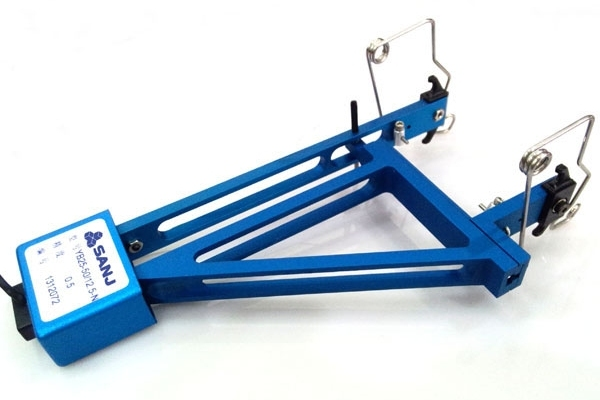Specify total elongation strength

With the continuous progress of science and technology and the development of engineering field, the testing of mechanical properties of materials becomes more and more important. Among them, the total elongation strength is a key parameter, which can not only evaluate the strength and toughness of materials, but also play an important role in many engineering designs and material selection. In this paper, we will discuss the significance of total elongation strength in mechanical property testing and some common testing methods.

The significance of total elongation strength

The total elongation strength is the maximum stress value that the material can withstand under the action of force. It directly reflects the strength and toughness of the material, and is an important index for evaluating material properties. By measuring the total elongation strength of a material, we can find out whether the material is able to maintain its structural integrity and stability under external forces. This is critical for various engineering fields such as aerospace, automotive manufacturing, and construction engineering.Commonly used mechanical property test methods

1. Tensile test

The tensile test is a common test method used to determine the properties of a material under tensile loading. By applying tensile force and gradually increasing the force on the material, we can measure the deformation and breaking point of the material to determine its total elongation strength. This method is simple and easy to use and is widely used in various fields.

2. Compression test

Compression test is another commonly used test method to determine the properties of a material under compressive loading. By applying pressure, we can observe the behavior of the material during compression and calculate the total elongation strength. Compression test is very important for some specific application scenarios such as in construction materials and metal engineering.

3. Bending test

Bending test is a method to test the behavior of a material under bending loading. By applying a bending force, we can observe how the material behaves during bending and calculate its total elongation strength. Bending test is mainly used to evaluate the toughness and durability of materials, and it is very important for some structures and materials selection that need to withstand bending force.Conclusion

Total elongation strength, as an important parameter for mechanical property testing, plays a crucial role in engineering design and material selection. By using some common testing methods, such as tensile, compression and bending tests, we can accurately assess the total elongation strength of materials and provide strong support and reference for engineering practices in various fields. Therefore, it is of great significance to strengthen the research and application of mechanical property testing to promote the development of engineering technology and enhance the performance of materials.

Related experiments